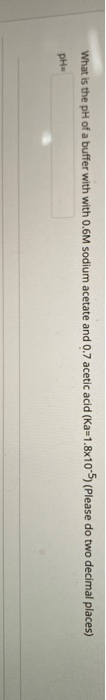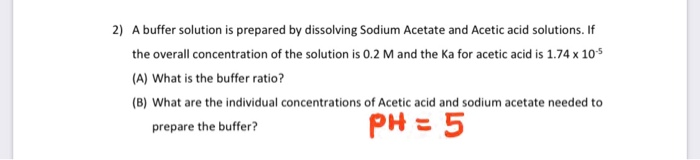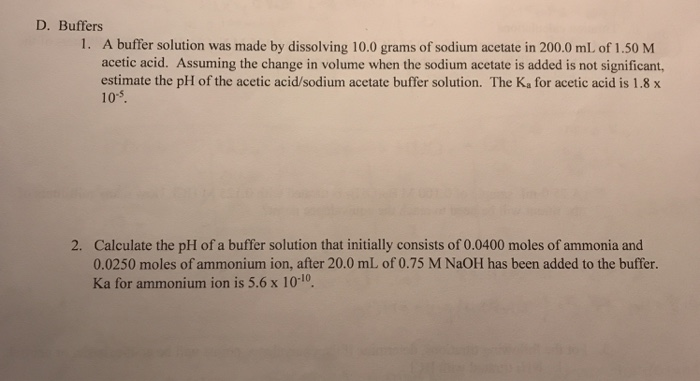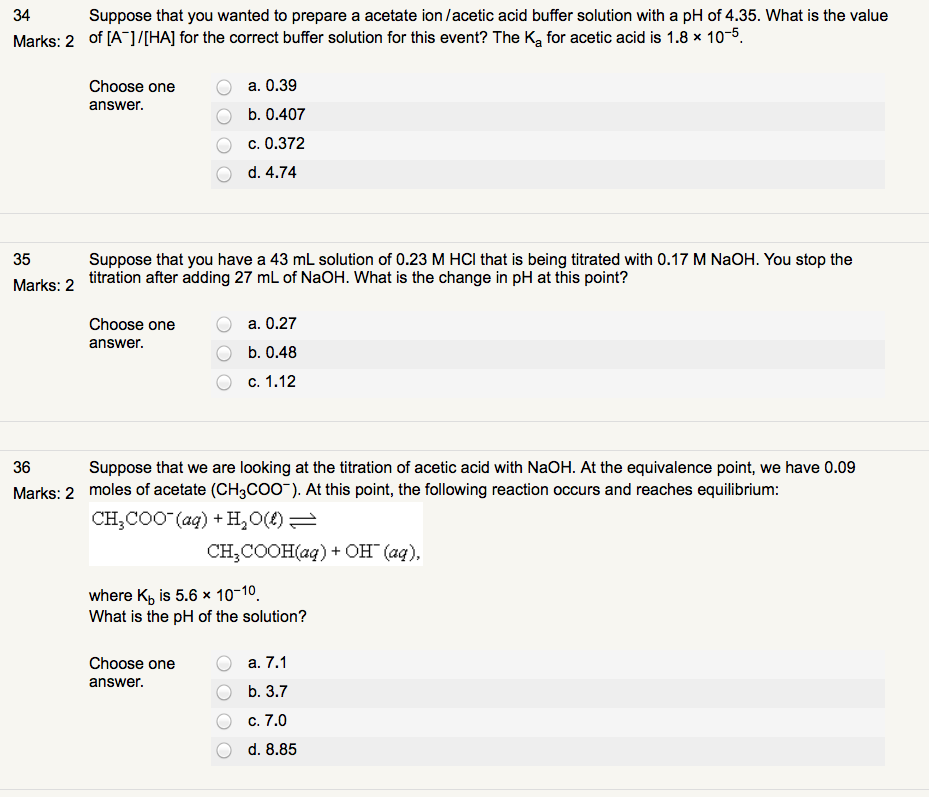Homework Help Question & Answers

# What is the pH of a 0.3M acetic acid and 0.3M sodium acetate buffer solution after...

What is the pH of a 0.3M acetic acid and 0.3M sodium acetate buffer solution after adding 0.1M NaOH? (Ka = 1.75*10^-5)

#### Homework Answers

Answer #1

Using H-H equation,
pH = pKa + log[sodium acetate]/[acetic acid]
pKa = -logKa = -log(1.75 × 10^-5) = 4.73282827
After adding 0.1 M NaOH,
[sodium acetate] = (0.3 + 0.1) M = 0.4 M
[Acetic acid] = (0.3 - 0.1) M = 0.2 M
pH = 4.73282827 + log (0.4/0.2)
4.73282827 + 0.301029996 = 5.03385827 = 5.03
pH = 5.03

Know the answer?
Your Answer:

#### Post as a guest

Your Name:

What's your source?

#### Earn Coin

Coins can be redeemed for fabulous gifts.

Not the answer you're looking for? Ask your own homework help question. Our experts will answer your question WITHIN MINUTES for Free.
Similar Homework Help Questions
• ### A buffer solution is 0.78 M in acetic acid and 0.22 M in sodium acetate. Calculate...

A buffer solution is 0.78 M in acetic acid and 0.22 M in sodium acetate. Calculate the solution pH after adding 0.80 g of solid NaOH to 100.0 mL of the buffer solution. Ka of acetic acid is 1.8  10−5 . Assume negligible volume change.

• ### a buffer solution of pH =5.30 can be prepared by dissolving acetic acid and sodium acetate...

a buffer solution of pH =5.30 can be prepared by dissolving acetic acid and sodium acetate in water. How many moles of sodium acetate must be added to 1 L of 0.25 M acetic acid to prepare the buffer? Ka(CH3COOH)=1.8 x 10^-5

• ### For a buffer made from sodium acetate and acetic acid, the Ka of acetic acid is...

For a buffer made from sodium acetate and acetic acid, the Ka of acetic acid is 1.8E-5. What mass of sodium acetate (NaCH3CO2) must be added to 2.50 L of 0.68 M acetic acid to make a buffer solution with pH = 5.75? The answer should be in two significant figures.

• ### A buffer solution of 0.200M for both acetic acid, HC2H3O2 and sodium acetate, NaC2H3O2, has a...

A buffer solution of 0.200M for both acetic acid, HC2H3O2 and sodium acetate, NaC2H3O2, has a pH of 4.74. If a solution of 0.0400M HCl is added, what is the new pH of the solution? (the buffer acid used has a Ka = 1.8 x 10-5)

• ### What is the pH of a buffer with with 0.6M sodium acetate and 0.7 acetic acid...What is the pH of a buffer with with 0.6M sodium acetate and 0.7 acetic acid (Ka=1.8x10-5) (Please do two decimal places) PH

• ### 2) A buffer solution is prepared by dissolving Sodium Acetate and Acetic acid solutions. If the...2) A buffer solution is prepared by dissolving Sodium Acetate and Acetic acid solutions. If the overall concentration of the solution is 0.2 M and the Ka for acetic acid is 1.74 x 105 (A) What is the buffer ratio? (B) What are the individual concentrations of Acetic acid and sodium acetate needed to prepare the buffer? PH = 5 Can you please write down any assumptions needed for this particular problem? My Professor needs to see thought process. Thank...

• ### D. Buffers 1. A buffer solution was made by dissolving 10.0 grams of sodium acetate in...D. Buffers 1. A buffer solution was made by dissolving 10.0 grams of sodium acetate in 200.0 mL of 1.50 M acetic acid. Assuming the change in volume when the sodium acetate is added is not significant, estimate the pH of the acetic acid/sodium acetate buffer solution. The K, for acetic acid is 1.8 x 105. 2. Calculate the pH of a buffer solution that initially consists of 0.0400 moles of ammonia and 0.0250 moles of ammonium ion, after 20.0...

• ### A buffer solution contains 0.120M acetic acid and 0.150M sodium acetate

A buffer solution contains 0.120M acetic acid and 0.150M sodium acetate. a. How many moles of acetic acid and sodium acetate are present in 50.0 ml of solution? b. if we add 5.55 mL of 0.092M NaOH to the solution in part (a) how many moles of acetic acid , sodium acetate, and NaOH will be present after the reaction is finished? c. if we add 0.50 ml of 0.087 M HCI to the solution in part (a), how many...

• ### Buffer Problem! (Sodium Acetate + Acetic Acid)

<p>Your goal is to prepare 100.00mL of a buffer with a pH of 3.500. You have in your stock: 1.00M acetic acid and 1.00M sodium acetate. The Ka of acetic acid =1.76 x 10^-5. The total ion concentration (initial concentration of sodium acetate + initial concentration of acetic acid) must be 0.100 M. How would you go aboutcreating this buffer?</p>

• ### Suppose that you wanted to prepare a acetate ion/acetic acid buffer solution with a pH of...Suppose that you wanted to prepare a acetate ion/acetic acid buffer solution with a pH of 4.35. What is the value 34 Marks: 2 off [A l/IHA) for the correct buffer solution for this event? The Ka for acetic acid is 1.8 x 10 5. a. 0.39 Choose one answer. b. 0.407 c. 0.372 d. 4.74 Suppose that you have a 43 mL solution of 0.23 M HCl that is being titrated with 0.17 M NaOH. You stop the 35...

Free Homework App

Scan Your Homework
to Get Instant Free Answers
Need Online Homework Help?

Get Answers For Free
Most questions answered within 3 hours.